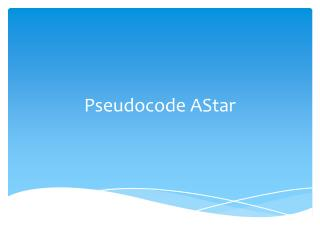DownloadDownload PresentationPseudocode AStar

# Pseudocode AStar

Download Presentation## Pseudocode AStar

- - - - - - - - - - - - - - - - - - - - - - - - - - - E N D - - - - - - - - - - - - - - - - - - - - - - - - - - -
##### Presentation Transcript

1. PseudocodeAStar

2. Heuristics - how to determine what's a good next step? As mentioned already, the A* algorithm depends on evaluating the best next step in searching for a path. This is done by evaluating each of the possible next steps against a heuristic to give a value that can be used to sort the list and hence determine the most likely next step. As you can imagine this makes choosing a good heuristic for your map and game really important to getting good path finding performance.

3. Euclidean Distance The most obvious way to determine the best step is to always pick the step that is the closest to the target. This isn't always perfect for environments with a lot of obstacles or that are maze like but it does provide a simple heuristic to understand. It would look like: dx = targetX - currentX; dy = targetY - currentY; heuristic = sqrt((dx*dx)+(dy*dy)); Remembering that the heuristic is evaluated frequently during the path finding process we can see that this may not always be a good idea. That sqrt() call is (on most hardware) expensive.

4. Manhattan Distance Another common approach is to replace absolute distance with "Manhattan Distance". This is an approximation of the distance between two points based on adding the horizontal distance and vertical distances rather than computing the exact difference. That would look like this: dx = abs(targetX - currentX); dy = abs(targetY - currentY); heuristic = dx+dy;

5. Pseudo Code The A* algorithm works like this: • At initialization add the starting location to the open list and empty the closed list • While there are still more possible next steps in the open list and we haven't found the target: • Select the most likely next step (based on both the heuristic and path costs) • Remove it from the open list and add it to the closed • Consider each neighbor of the step. For each neighbor: • Calculate the path cost of reaching the neighbor • If the cost is less than the cost known for this location then remove it from the open or closed lists (since we've now found a better route) • If the location isn't in either the open or closed list then record the costs for the location and add it to the open list (this means it'll be considered in the next search). Record how we got to this location The loop ends when we either find a route to the destination or we run out of steps. If a route is found we back track up the records of how we reached each location to determine the path.